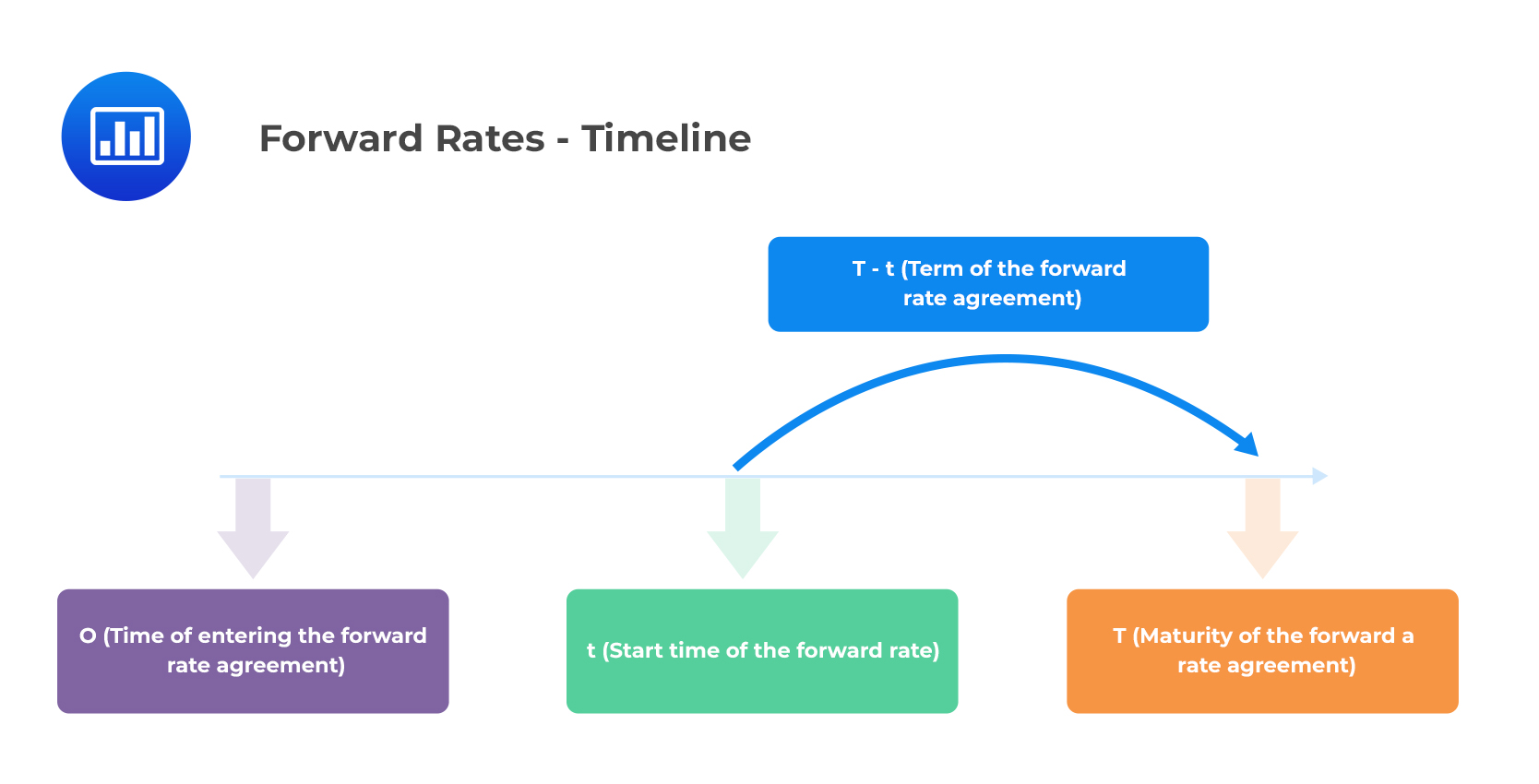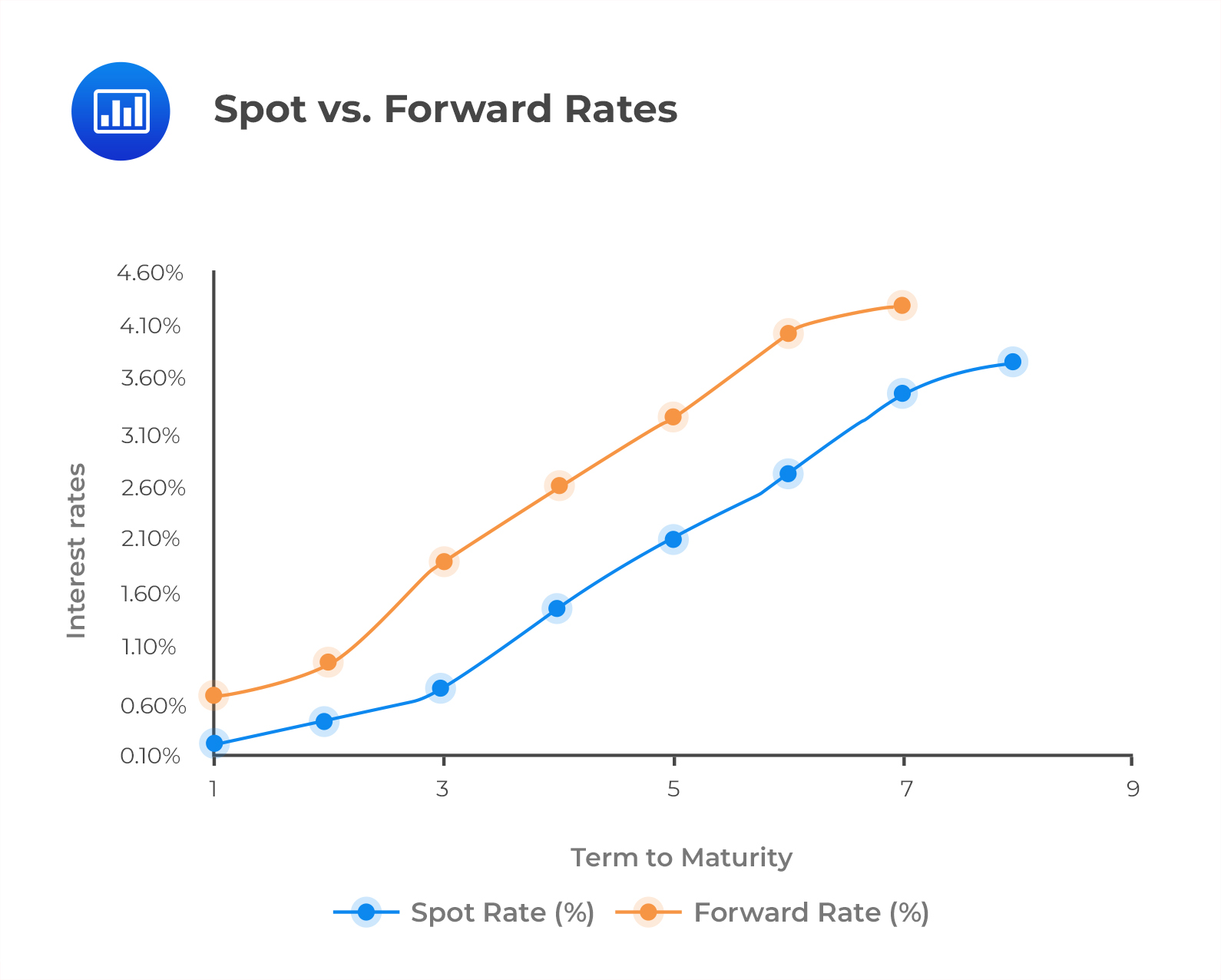# Spot Rates and Forward Rates

This reading will establish how interest rates and prices of bonds for different maturities are related.

## Spot Rates

#### Solution

$$P\left(T\right)=\frac{1}{\left(1+S\left(T\right)\right)^T}$$

The 10% interest rate is an example of a spot rate.

\begin{align*} P\left(2\right) &=\frac{100}{\left(1+0.10\right)^2} \\ P\left(2\right) &=82.64 \end{align*}

## Forward Rates

The forward rate $$f(0, t, T)$$ is the annualized interest rate payable on a loan, which is agreed upon today, starting at time $$t$$, to be repaid at maturity $$T$$.

In this case:

• $$0$$ is the time at which the forward rate agreement is entered.
• $$t$$ is the start time of the forward rate.
• $$T$$ is the maturity of the agreement.

This can be shown in the following timeline.$$F(0,t,T)$$ is the forward price (discount factor) of a zero-coupon bond, agreed upon at time 0, filled at time $$t$$, and maturing at time $$T$$. It is expressed mathematically as:

$$F\left(0, t, T\right)=\frac{1}{\left[1+f\left(0,t,T\right)\right]^{T-t}}$$

This can also be written as:

$$F\left(t,T-t\right)=\frac{1}{\left[1+f\left(t,T-t\right)\right]^{T-t}}$$

A forward curve is a graph showing the relationship between the forward rates and the related terms to maturity. The following figure illustrates both the forward curve and the spot curve.Notice that the forward curve lies above the spot curve for an upward sloping spot curve. Conversely, the forward curve will lie below the spot curve for a downward sloping spot curve. This will be discussed later in the forward rate model.

## Yield to Maturity

The yield to maturity (YTM) is the discount rate that equates the present value of future bond payments (includes coupons and the par value) to the bond’s market price.

In other words, YTM is the expected rate of return on a bond if:

1. The bond is held to maturity.
2. The bold does not default.
3. Reinvestment of the bond and all coupons is executed at the original yield to maturity.

If the spot rate curve of a coupon-paying bond is flat, the YTM will be the same as the spot rate. Also, note that the YTM of a zero-coupon bond is equal to the spot rate.

1. 8%.
2. 9%.
3. 47%.

#### Solution

\begin{align*} P\left(T\right)&=\frac{1}{\left(1+S\left(T\right)\right)^T} \\ \\ 34,029 &=\frac{50,000}{\left(1+S_5\right)^5} \\ \\ S_5 &=8\% \end{align*}

N/B: The YTM of a zero-coupon bond is equal to the spot rate.

Reading 28: The Term Structure and Interest Rate Dynamics

LOS 28 (a) Describe relationships among spot rates, forward rates, yield to maturity, expected and realized returns on bonds, and the shape of the yield curve.

Shop CFA® Exam Prep

Offered by AnalystPrepLevel I
Level II
Level III
All Three Levels
Featured Shop FRM® Exam PrepFRM Part I
FRM Part II
FRM Part I & Part II
Learn with Us

Subscribe to our newsletter and keep up with the latest and greatest tips for success
Shop Actuarial Exams PrepExam P (Probability)
Exam FM (Financial Mathematics)
Exams P & FMGMAT® Complete CourseDaniel Glyn
2021-03-24
I have finished my FRM1 thanks to AnalystPrep. And now using AnalystPrep for my FRM2 preparation. Professor Forjan is brilliant. He gives such good explanations and analogies. And more than anything makes learning fun. A big thank you to Analystprep and Professor Forjan. 5 stars all the way!michael walshe
2021-03-18
Professor James' videos are excellent for understanding the underlying theories behind financial engineering / financial analysis. The AnalystPrep videos were better than any of the others that I searched through on YouTube for providing a clear explanation of some concepts, such as Portfolio theory, CAPM, and Arbitrage Pricing theory. Watching these cleared up many of the unclarities I had in my head. Highly recommended.Nyka Smith
2021-02-18
Every concept is very well explained by Nilay Arun. kudos to you man!2021-02-13